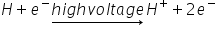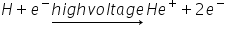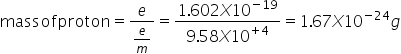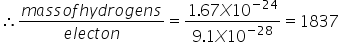# Discovery of Proton

Proton was discovered by E. GoldStein. He using a perforated cathode , when high voltage is passed  though a gas under reduced pressure then source rays travel in the opposite direction to the cathode rays. These rays are produced at the anode so called as anode rays. These rays pass through the holes of the cathode hence called as positive rays or canal rays (because they passed through holes in the cathode)

Origin of anode rays-

High energy electrons moving from the cathode ionize the atom / molecule of the gas.

Moving electron knocks another electron out  of a hydrogen atom, thus leaving a positive charge in hydrogen .thus  result out hydrogen ions constitute the positive rays

eq.(Helium Ion)

Na + e- → Na+ +2e-

(Sodium ion)

Proof of anode rays- some as that of cathode rays. But anode rays are positive charge particle charge/mass ratio is found to be different depend upon the nature of gas taken in discharge tube. Mass of the particle to be different for different gases taken W.Wein found that e/m value is the lightest when hydrogen gas was used + concluded that hydrogen atom is lightest.

Calculation of mass of proton-

Charge = same as that of electron

(regarded as positive charge)

e=1.602 x 10-19C

e/m = 9.58 x 104 C/gthis mass is nearly same as that of hydrogen atom( thus mass of proton is abort 1837 times to the mass of  electron)

Related Keywords